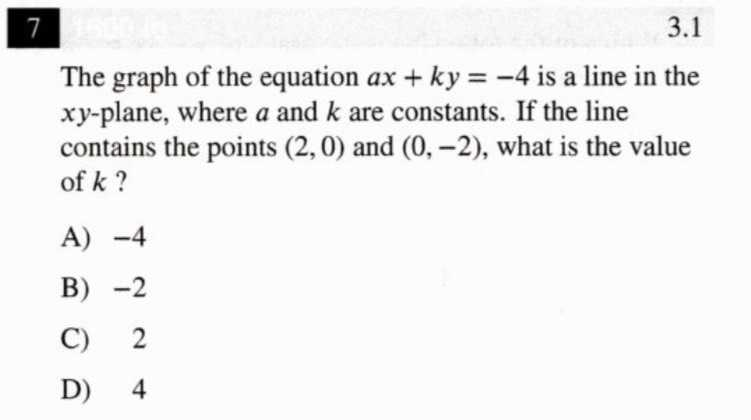### ¿Todavía tienes preguntas de matemáticas?

Pregunte a nuestros tutores expertos
Algebra
Pregunta$$7$$ The graph of the equation $$a x + k y = - 4$$ is a line in the $$x y$$ -plane, where $$a$$ and $$k$$ are constants. If the line contains the points $$( 2,0 )$$ and $$( 0 , - 2 ) ,$$ what is the value of $$k ?$$ A) $$- 4$$ B) $$- 2$$ C) $$2$$ D) $$4$$